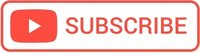# Python, check if a number is Prime

## What is a prime number?

A prime number is divisible only by itself and 1. Prime numbers are important in cryptography (such as RSA cryptosystem) because the security of many encryption algorithms is based on the fact that it is very fast to multiply two large prime numbers and get the result, while it is extremely expensive in terms of execution time to do the reverse.

For example, if you have a “public key” consisting of a product of two large primes used to encrypt a message, and a “secret key” consisting of those two primes used to decrypt the message, You can make the public key public, and everyone can use it to encrypt messages to you, but only you know the prime factors and can decrypt the messages. Everyone else would have to factor the number, which takes too long to be practical, given the current state of the art of number theory.

# Check if a number is Prime Python solutions

## A clean solution that uses list comprehensions

# A clean solution that uses list comprehensions

number = int(input("Enter the number\n"))
# excludes the number itself and 1, both of which the number is divisible
divisors = [i for i in range(2, number) if number % i == 0]

if len(divisors) == 0:
print(number, "is prime\n")
else:
print(number, "is not prime")
print("Its divisors are:", divisors)


## Another classic solution

number = int(input("Enter the number\n"))

divisors = []
for i in range(2, number):  # excludes the number itself and 1, both of which the number is divisible
if number % i == 0:
divisors.append(i)

if len(divisors) == 0:
print(number, "is prime\n")
else:
print(number, "is not prime")
print("Its divisors are:", divisors)


#### Hello there!

I hope you find this post useful!

I'm Mihai, a programmer and online marketing specialist, very passionate about everything that means online marketing, focused on eCommerce.

If you have a collaboration proposal or need helps with your projects feel free to contact me. I will always be glad to help you!